# Equivalent Ratios and Tables Unit 1 Lesson 3

• Slides: 28Equivalent Ratios and Tables Unit 1 Lesson 3 Math 6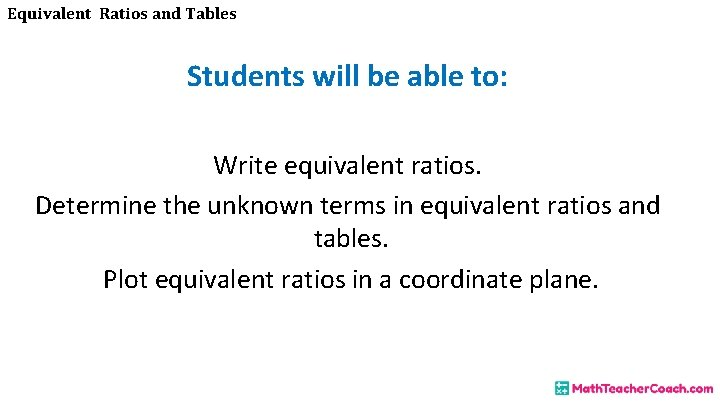Equivalent Ratios and Tables Students will be able to: Write equivalent ratios. Determine the unknown terms in equivalent ratios and tables. Plot equivalent ratios in a coordinate plane.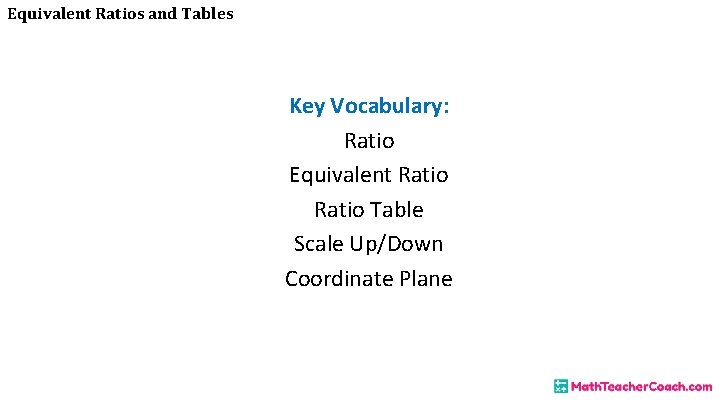Equivalent Ratios and Tables Key Vocabulary: Ratio Equivalent Ratio Table Scale Up/Down Coordinate Plane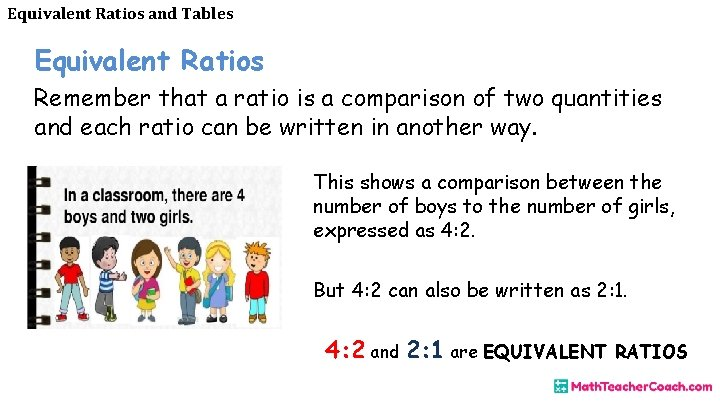Equivalent Ratios and Tables Equivalent Ratios Remember that a ratio is a comparison of two quantities and each ratio can be written in another way. This shows a comparison between the number of boys to the number of girls, expressed as 4: 2. But 4: 2 can also be written as 2: 1. 4: 2 and 2: 1 are EQUIVALENT RATIOSEquivalent Ratios and Tables How do we write equivalent ratios? Equivalent ratios can be determined by SCALING UP or SCALING DOWN a ratio.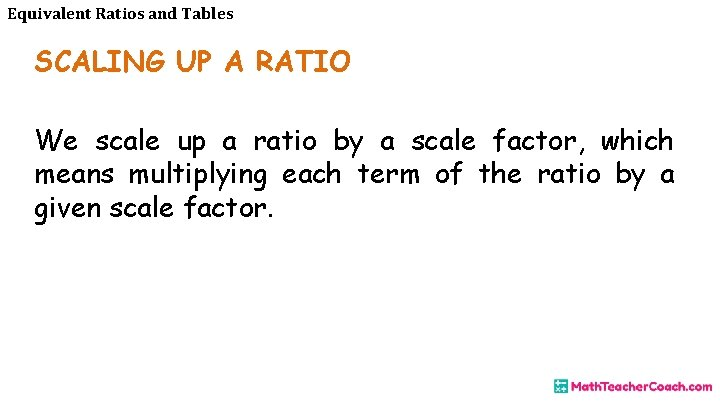Equivalent Ratios and Tables SCALING UP A RATIO We scale up a ratio by a scale factor, which means multiplying each term of the ratio by a given scale factor.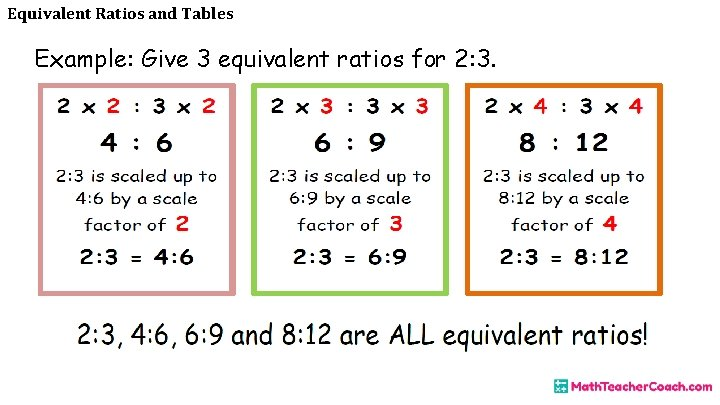Equivalent Ratios and Tables Example: Give 3 equivalent ratios for 2: 3.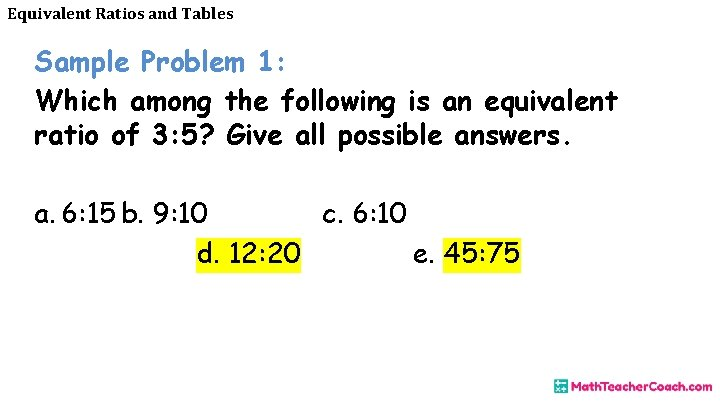Equivalent Ratios and Tables Sample Problem 1: Which among the following is an equivalent ratio of 3: 5? Give all possible answers. a. 6: 15 b. 9: 10 c. 6: 10 d. 12: 20 e. 45: 75Equivalent Ratios and Tables SCALING DOWN A RATIO We scale down a ratio by a scale factor, which means dividing each term of the ratio by a given scale factor.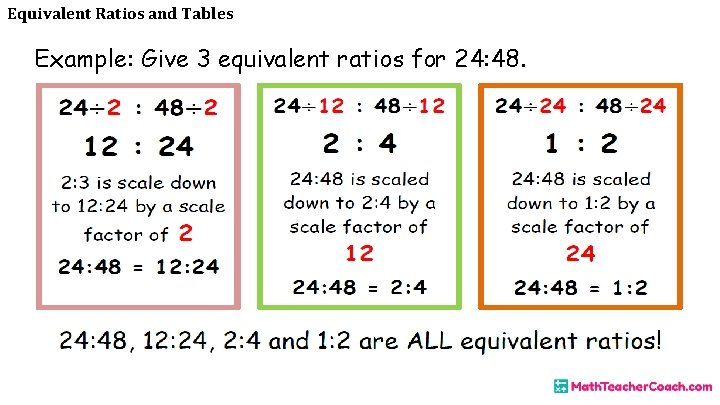Equivalent Ratios and Tables Example: Give 3 equivalent ratios for 24: 48.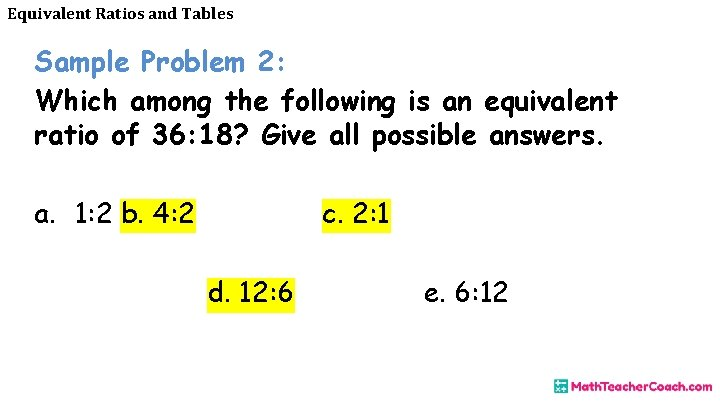Equivalent Ratios and Tables Sample Problem 2: Which among the following is an equivalent ratio of 36: 18? Give all possible answers. a. 1: 2 b. 4: 2 c. 2: 1 d. 12: 6 e. 6: 12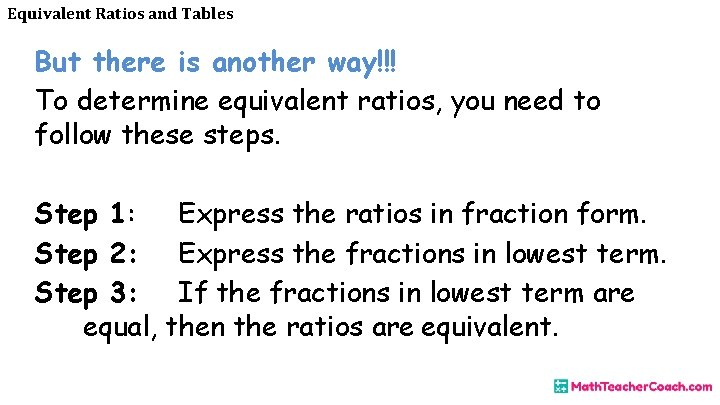Equivalent Ratios and Tables But there is another way!!! To determine equivalent ratios, you need to follow these steps. Step 1: Express the ratios in fraction form. Step 2: Express the fractions in lowest term. Step 3: If the fractions in lowest term are equal, then the ratios are equivalent.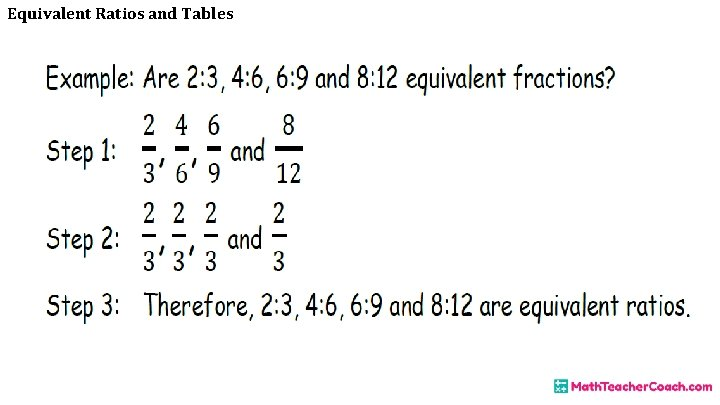Equivalent Ratios and TablesEquivalent Ratios and Tables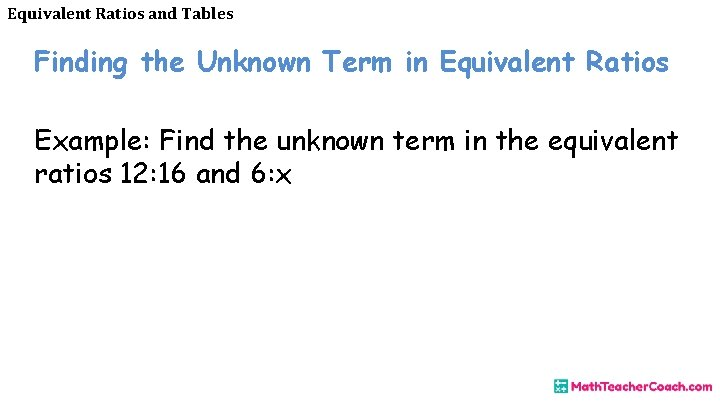Equivalent Ratios and Tables Finding the Unknown Term in Equivalent Ratios Example: Find the unknown term in the equivalent ratios 12: 16 and 6: x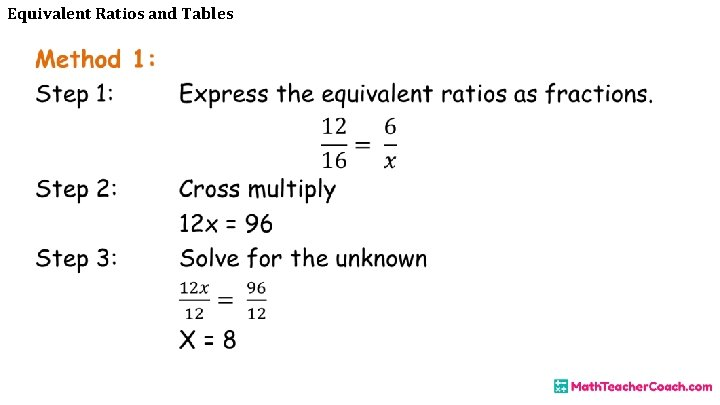Equivalent Ratios and Tables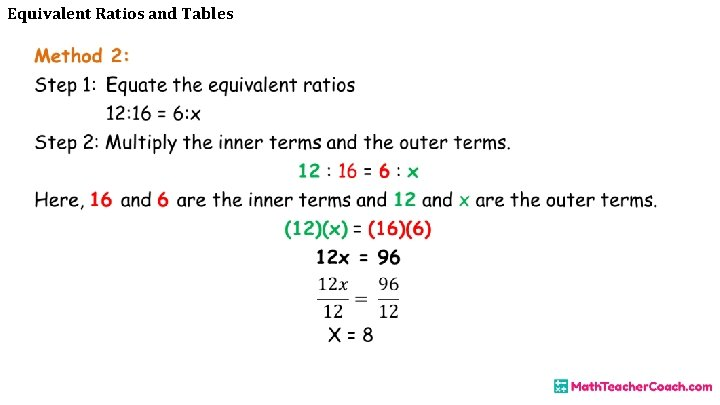Equivalent Ratios and TablesEquivalent Ratios and Tables Sample Problem 4: The ratio of boys to girls in a photography club is 3: 4, If there are 12 boys, how many girls are there?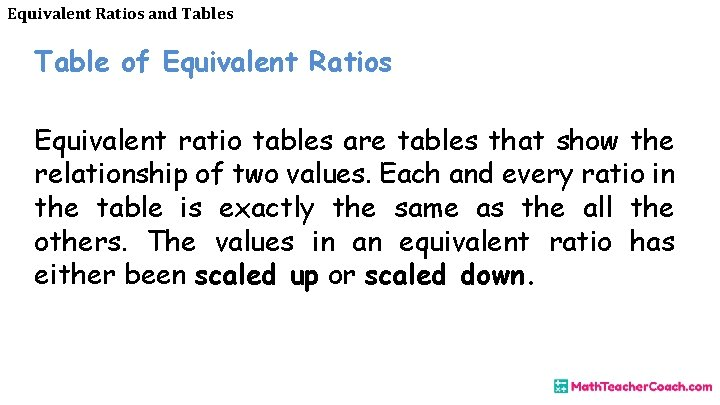Equivalent Ratios and Tables Table of Equivalent Ratios Equivalent ratio tables are tables that show the relationship of two values. Each and every ratio in the table is exactly the same as the all the others. The values in an equivalent ratio has either been scaled up or scaled down.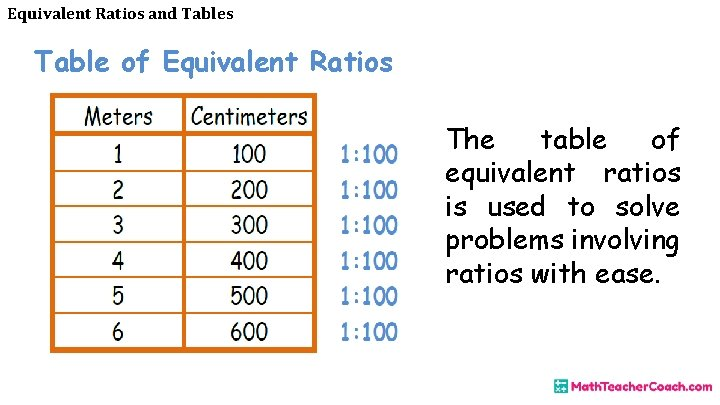Equivalent Ratios and Tables Table of Equivalent Ratios The table of equivalent ratios is used to solve problems involving ratios with ease.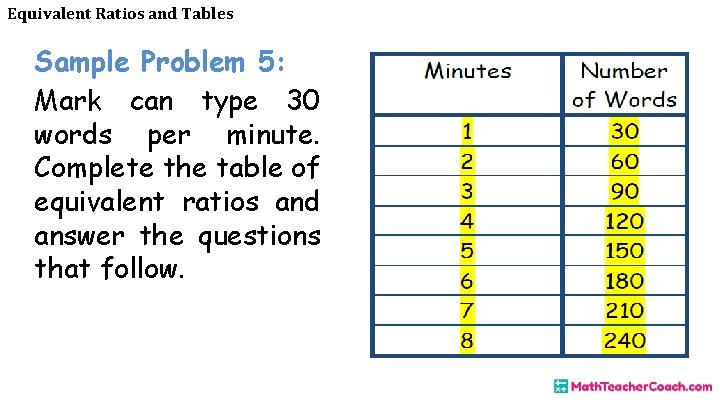Equivalent Ratios and Tables Sample Problem 5: Mark can type 30 words per minute. Complete the table of equivalent ratios and answer the questions that follow.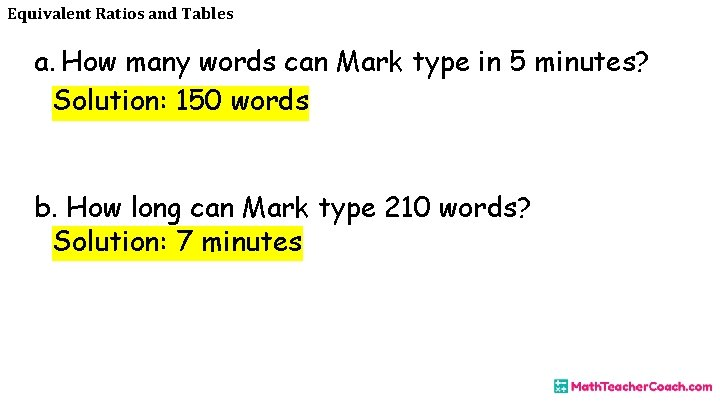Equivalent Ratios and Tables a. How many words can Mark type in 5 minutes? Solution: 150 words b. How long can Mark type 210 words? Solution: 7 minutes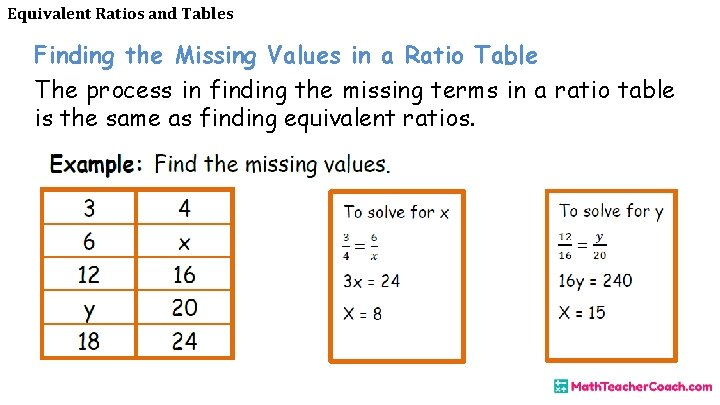Equivalent Ratios and Tables Finding the Missing Values in a Ratio Table The process in finding the missing terms in a ratio table is the same as finding equivalent ratios.Equivalent Ratios and Tables Ratios on Coordinate Plane The pairs of values in the table of equivalent ratio can be plotted in a coordinate plane. The graph should be a straight line. Example: Plot the table of equivalent ratios in a coordinate plane.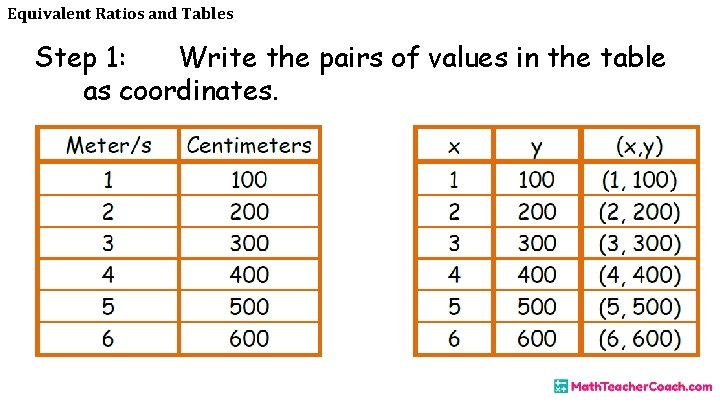Equivalent Ratios and Tables Step 1: Write the pairs of values in the table as coordinates.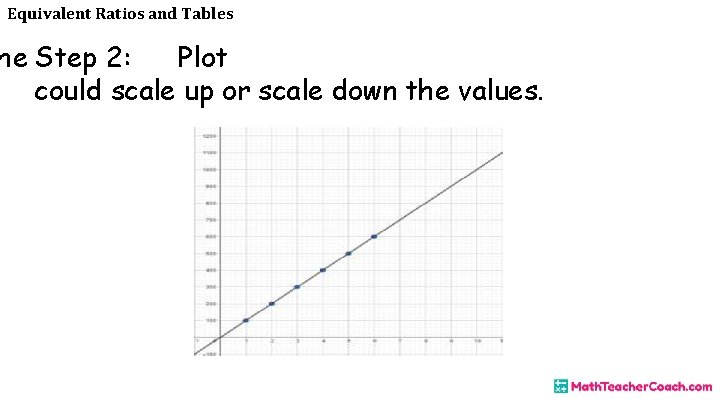Equivalent Ratios and Tables he Step 2: Plot could scale up or scale down the values.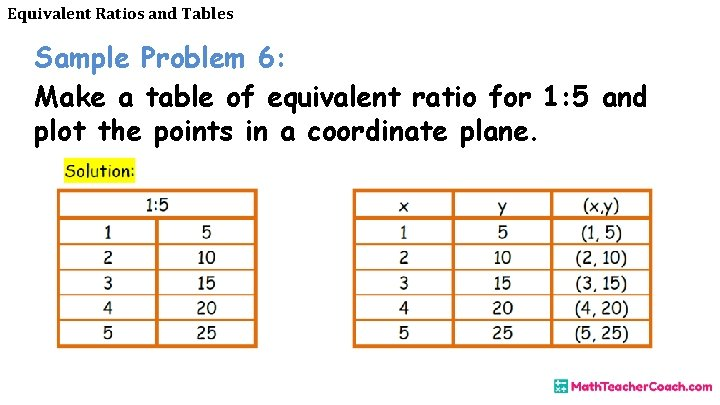Equivalent Ratios and Tables Sample Problem 6: Make a table of equivalent ratio for 1: 5 and plot the points in a coordinate plane.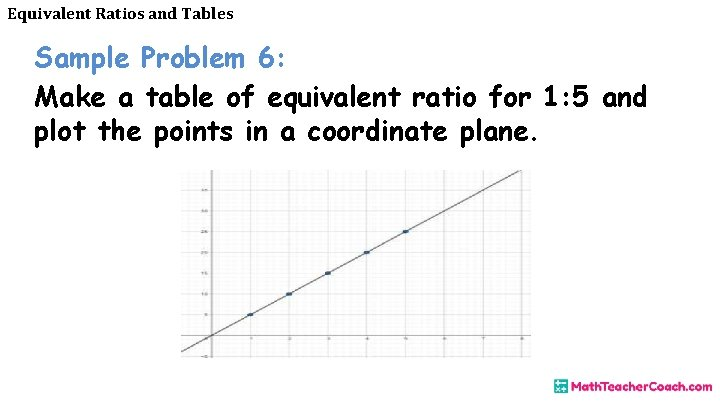Equivalent Ratios and Tables Sample Problem 6: Make a table of equivalent ratio for 1: 5 and plot the points in a coordinate plane.